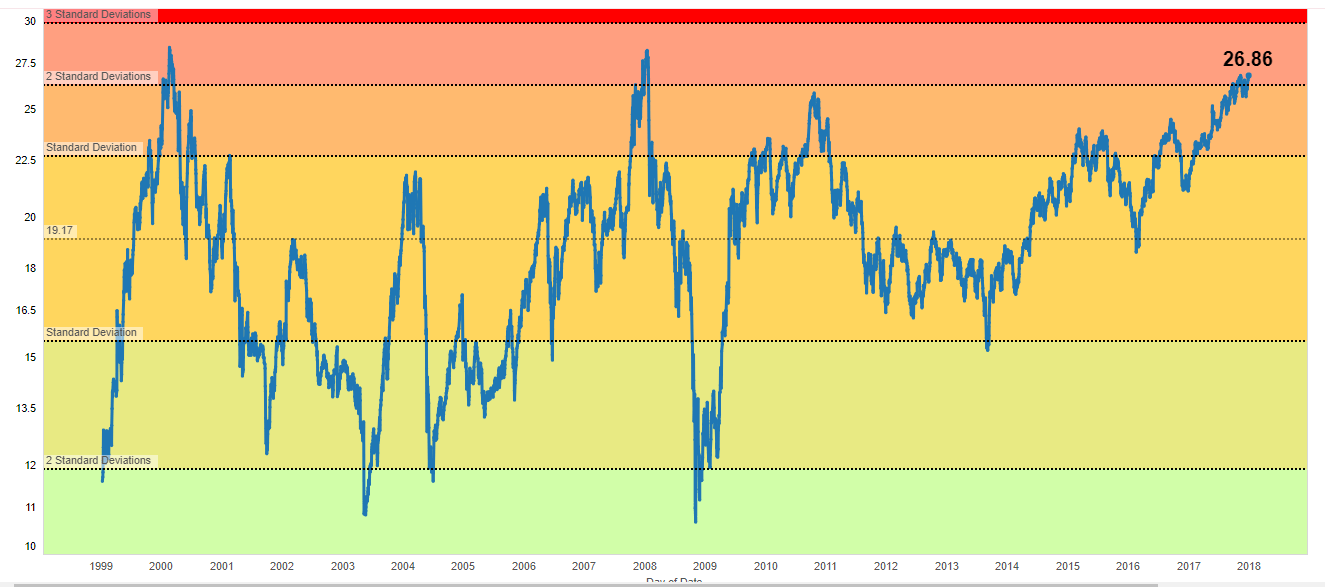## December 24, 2017

### Nifty PE Ratio Analysis – Are we at the Tipping Point?

Current PE Ratio Metric at 26.86 is little above the 2 standard deviation.

So how does it matters to a common investor? In simple statistical terms – Approximately 95% of the area of a normal distribution is within two standard deviations of the mean. i.e in simple terms 95% probability that Nifty is highly overvalued in normal distribution terms with mean reversion tendency is relatively high. Year 2000 and 2008 are the other periods where Nifty crossed 2 standard deviation where the end result is not a good outcome for the investors with short term or medium term horizon.Read more at http://www.marketcalls.in/fundamentals/nifty-pe-ratio-analysis-tipping-point.html